# Need help creating Tema MACD Crossover

#### dmaffo

##### Member
VIP
I was trying to copy and paste things but, you guys are the pros. Can someone help me create this or direct me so I can learn how to do it?
Thanks.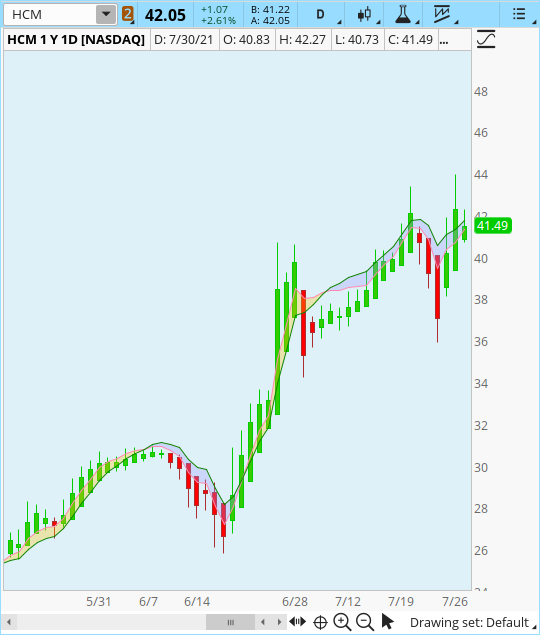Ruby:
``````# ########################################################
# TOS TEMA DEMA cloud
plot TEMA1 = TEMA("price" = close, "length" = 20);
plot DEMA2 = DEMA("price" = close, "length" = 9);
# ########################################################``````
Here is my short cut that I use whenever I need to understand what the syntax and inputs are:
@dmaffo

Awesome, I cant wait to get home and start messing aroumd with it. Thank you

Appreciate the help but I havent been able to make anything work yet. Would love to get a bit more help on this one if possible.
Thanks

MerryDay,
My bad, I was simply trying to learn how to do this was all, and I at the moment still cant figure it out. I got the script you posted and it was so short and easy I cant believe it. Sincerely thank you.

•MerryDay
How do I convert lines crossing so they turn green or red depending on above or below each other?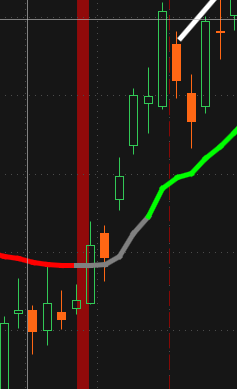Code:
``Line1.AssignValueColor(if Line1 is greater than Line2 then Color.GREEN else color.red);``

Instead of spending days on this let me share with you what I have done so far copy and pasting things. I cant get the inputs and options to work right, 100%. The clouds arent working according to which "plots" I select. If I want to show clouds ONLY on the selected studies is that possible?
Also the line colour of lets say TEMA and PMACDeq corresponding to their relative positions to each other.

Here is what I have so far:
Ruby:
``````declare upper;

# TEMA
input price = close;
input length = 10;

def ema1 = ExpAverage(price, length);
def ema2 = ExpAverage(ema1, length);
def ema3 = ExpAverage(ema2, length);

def TEMA = 3 * ema1 - 3 * ema2 + ema3;;

plot TEMA1 = TEMA("price" = close, "length" = 10);
plot DEMA2 = DEMA("price" = close, "length" = 10);

#input price = close;
input fastLength = 12;
input slowLength = 26;
input MACDLength = 9;
input AverageType = {SMA, default EMA};
input MACDLevel = 0.0;

def fastCoeff = 2 / (1 + fastLength);
def slowCoeff = 2 / (1 + slowLength);
def prevFastEMA = ExpAverage(price, fastLength);
def prevSlowEMA = ExpAverage(price, slowLength);
def prevFastSMA = Average(price, fastLength);
def prevSlowSMA = Average(price, slowLength);
def prevDiff;
def crossDiff;
def denominator;

plot PMACDeq;
plot PMACDlevel;
plot MA_PMACDeq;
plot PMACDsignal;

switch (AverageType) {
case SMA:
crossDiff = slowLength * price[fastLength] - fastLength * price[slowLength];
denominator = slowLength - fastLength;
prevDiff = prevFastSMA - prevSlowSMA;
PMACDlevel = (crossDiff + fastLength * slowLength * (MACDLevel - prevDiff)) / denominator;
if IsNaN(price) {
PMACDeq = Double.NaN;
MA_PMACDeq = Double.NaN;
} else {
PMACDeq = crossDiff / denominator;
MA_PMACDeq = Average(PMACDeq, MACDLength);
}
PMACDsignal = (crossDiff + fastLength * slowLength * (Average(prevDiff, MACDLength - 1) - prevDiff)) / denominator;
case EMA:
crossDiff = prevFastEMA * fastCoeff - prevSlowEMA * slowCoeff;
denominator = fastCoeff - slowCoeff;
prevDiff = prevFastEMA - prevSlowEMA;
PMACDeq = crossDiff / denominator;
PMACDlevel = (MACDLevel - prevDiff + crossDiff) / denominator;
MA_PMACDeq = ExpAverage(PMACDeq, MACDLength);
PMACDsignal = (ExpAverage(prevDiff, MACDLength) - prevDiff + crossDiff) / denominator;
}

PMACDeq.SetDefaultColor(GetColor(1));
MA_PMACDeq.SetDefaultColor(GetColor(8));
PMACDlevel.SetDefaultColor(GetColor(3));
PMACDsignal.SetDefaultColor(GetColor(5));

Last edited by a moderator:
•MerryDay
@dmaffo Very Nice! There were some housekeeping issues. There was ALOT of extraneous 'stuff' attempting to PLOT on the chart. PLOT statements are only for what is to be displayed on the chart. You only asked for 2 plots but the code had: 6 plots. Other than that I didn't have to touch anything. It works well. You might want to play w/ the 'length' settings. Sometimes, they need to be adjusted depending on the timeframe to be more responsive. Could you edit the top of your post, give your chart a name, explain to readers what each line represents and what you are looking for in this strategy?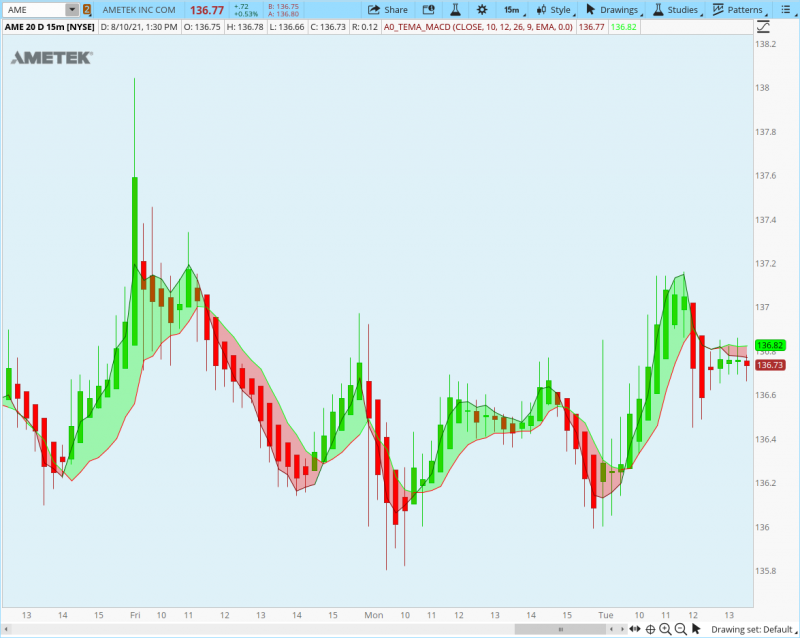Ruby:
``````declare upper;

# TEMA
input price = close;
input length = 10;
plot TEMA1 = TEMA("price" = close, "length" = 10);

#input price = close;
input fastLength = 12;
input slowLength = 26;
input MACDLength = 9;
input AverageType = {SMA, default EMA};
input MACDLevel = 0.0;

def fastCoeff = 2 / (1 + fastLength);
def slowCoeff = 2 / (1 + slowLength);
def prevFastEMA = ExpAverage(price, fastLength);
def prevSlowEMA = ExpAverage(price, slowLength);
def prevFastSMA = Average(price, fastLength);
def prevSlowSMA = Average(price, slowLength);
def prevDiff;
def crossDiff;
def denominator;

plot PMACDeq;

switch (AverageType) {
case SMA:
crossDiff = slowLength * price[fastLength] - fastLength * price[slowLength];
denominator = slowLength - fastLength;
prevDiff = prevFastSMA - prevSlowSMA;
if IsNaN(price) {
PMACDeq = Double.NaN;
} else {
PMACDeq = crossDiff / denominator;
}

case EMA:
crossDiff = prevFastEMA * fastCoeff - prevSlowEMA * slowCoeff;
denominator = fastCoeff - slowCoeff;
prevDiff = prevFastEMA - prevSlowEMA;
PMACDeq = crossDiff / denominator;
}
PMACDeq.AssignValueColor(if PMACDeq > TEMA1 then color.green else color.red) ;
TEMA1.AssignValueColor(if TEMA1  > PMACDeq then color.dark_green else color.dark_red) ;

Last edited:
I am loading it up as I am not able to simply open the link. Let me try open let you know how she looks.
What I am essentially doing is using these clouds on big screen monitor (8 screens total) behind me so that when I am looking at my preferred stocks I can tell which way the trend is going on bigger picture. I trade options and my personality gravitates more to a relative immediate positive position. I am not that well yet with swings or holding through initial red pull backs. So I want to use this to help me enter something that is moving in direction that would favor me whether I call or put the position.

•MerryDay
I changed the colors a bit so when I look back at monitor it looks like this: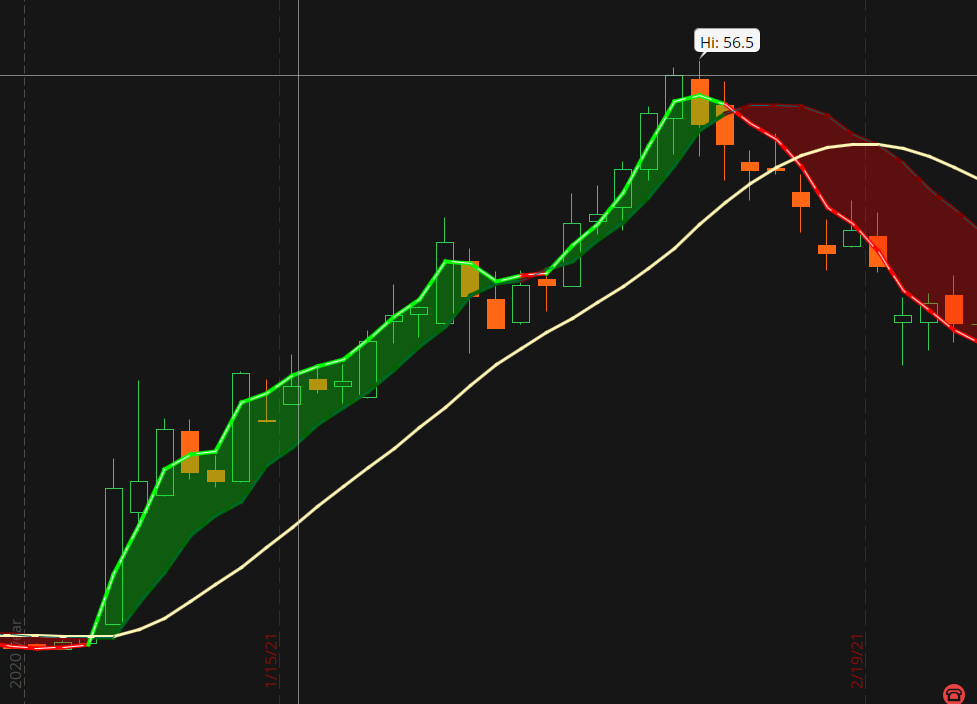•MerryDay
On the daily it will be easier for me to see the trend is all.
Thanks a whole bunch brother.

•MerryDay
I changed the colors a bit so when I look back at monitor it looks like this:@JoeSD All you need is the Study code posted above... Just Copy & Paste into a new Study and add it to whichever of your existing charts you desire, or add it to a new chart...

How do I add arrows @ crossover? I tried copy and pasting from another indicator but its shrinking the display.

declare lower;

input length = 7;
input signalLength = 10;
input averageType = AverageType.EXPONENTIAL;

def obv = reference OnBalanceVolume();

plot OBVM = MovingAverage(averageType, obv, length);
plot Signal = MovingAverage(averageType, OBVM, signalLength);

OBVM.SetDefaultColor(GetColor(8));
Signal.SetDefaultColor(GetColor(2));

def OBVM1 = MovingAverage(averageType, obv, length);
def Signal1 = MovingAverage(averageType, OBVM, signalLength);
def UpSignal = OBVM crosses above SIGNAL;
def DwnSignal = OBVM crosses below SIGNAL;

# Arrows
plot bullish = UpSignal;
bullish.SetPaintingStrategy(PaintingStrategy.BOOLEAN_ARROW_UP);
bullish.SetDefaultColor(Color.GREEN);
bullish.SetLineWeight(3);

plot bearish = DwnSignal;
bearish.SetPaintingStrategy(PaintingStrategy.BOOLEAN_ARROW_DOWN);
bearish.SetDefaultColor(Color.RED);
bearish.SetLineWeight(3);

##### End Code #####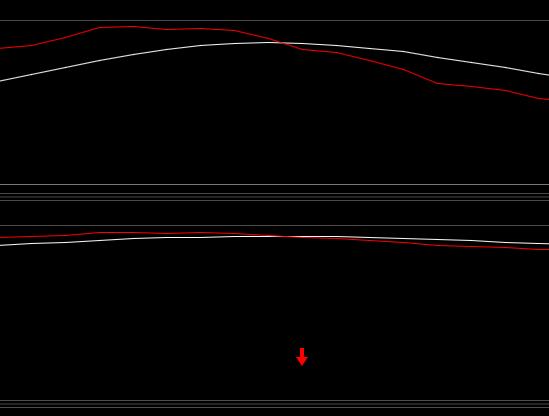notice top pic is the td indicator and I have only tried to add an arrow feature to it but its squishing the indicator to point its almost useless.

Creating Arrow Plots
When creating plots in thinkscript you not only have to define the logic of what you want plotted but you must also state WHERE on the candle or line that you want it plotted. (IE the low, high, open, close, of the candle).
So the syntax is if mycondition is true then low else double.Nan (print nothing)
Code:
``````plot bullish = if UpSignal  then low else double.Nan ;
plot bearish = if DwnSignal  then high else double.Nan ;``````
You 'may' need to take boolean logic out of the arrows

They being a bit difficult lol. I tried looking at MACD RSI to see if I could borrow some lines to get mine to work but still no go.
BUT I did get rid of the boolean and switched over to numerical.
PICS Below.
#TD Ameritrade IP Company, Inc. (c) 2020-2021
#

declare lower;

input length = 7;
input signalLength = 10;
input averageType = AverageType.EXPONENTIAL;

def obv = reference OnBalanceVolume();

plot OBVM = MovingAverage(averageType, obv, length);
plot Signal = MovingAverage(averageType, OBVM, signalLength);

OBVM.SetDefaultColor(GetColor(8));
Signal.SetDefaultColor(GetColor(2));

def OBVM1 = MovingAverage(averageType, obv, length);
def Signal1 = MovingAverage(averageType, OBVM, signalLength);
def UpSignal = OBVM crosses above SIGNAL;
def DwnSignal = OBVM crosses below SIGNAL;

# Arrows

plot bullish1 = if UpSignal then low else double.Nan ;
plot bearis1 = if DwnSignal then high else double.Nan ;

##### End Code #####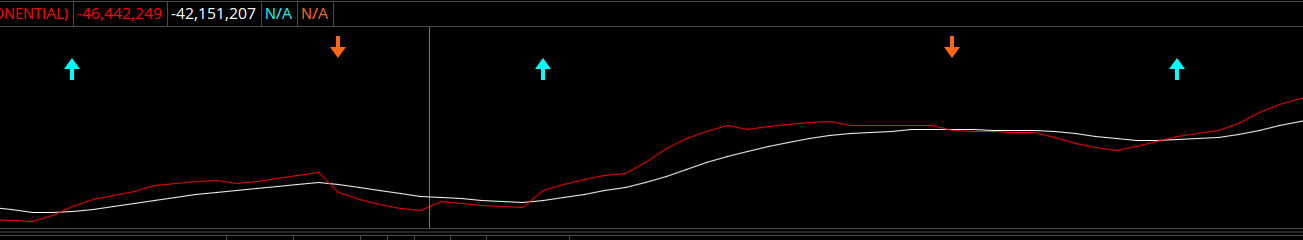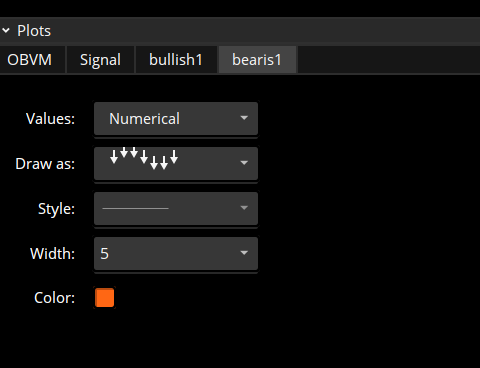OMGosh!Totally my fault~ I feel so bad that I added to your frustration... There are no low or high candles on a lower study SO OF COURSE, THE PLOTS I GAVE YOU DON'T WORK!

There is a OBVM so lets tell it to print on OBVM
Code:
``````plot bullish1 = if UpSignal then OBVM else double.Nan ;
plot bearis1 = if DwnSignal then OBVM else double.Nan ;``````

OMGosh!Totally my fault~ I feel so bad that I added to your frustration... There are no low or high candles on a lower study SO OF COURSE, THE PLOTS I GAVE YOU DON'T WORK!

There is a OBVM so lets tell it to print on OBVM
Code:
``````plot bullish1 = if UpSignal then OBVM else double.Nan ;
plot bearis1 = if DwnSignal then OBVM else double.Nan
[/QUOTE]``````

•MerryDay

### Not the exact question you're looking for?

87k+ Posts
199 Online## The Market Trading Game Changer

Join 2,500+ subscribers inside the useThinkScript VIP Membership Club
• Exclusive indicators
• Proven strategies & setups
• Private Discord community
• Exclusive members-only content
• 1 full year of unlimited support

What is useThinkScript?

useThinkScript is the #1 community of stock market investors using indicators and other tools to power their trading strategies. Traders of all skill levels use our forums to learn about scripting and indicators, help each other, and discover new ways to gain an edge in the markets.

How do I get started?

We get it. Our forum can be intimidating, if not overwhelming. With thousands of topics, tens of thousands of posts, our community has created an incredibly deep knowledge base for stock traders. No one can ever exhaust every resource provided on our site.

If you are new, or just looking for guidance, here are some helpful links to get you started.

What are the benefits of VIP Membership?